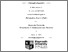# A Contribution to Metric Diophantine Approximation : the Lebesgue and Hausdorff Theories

Adiceam, Faustin (2014) A Contribution to Metric Diophantine Approximation : the Lebesgue and Hausdorff Theories. PhD thesis, National University of Ireland Maynooth.Previewmore...Add this article to your Mendeley library

## Abstract

This thesis is concerned with the theory of Diophantine approximation from the point of view of measure theory. After the prolegomena which conclude with a number of conjectures set to understand better the distribution of rational points on algebraic planar curves, Chapter 1 provides an extension of the celebrated Theorem of Duffin and Schaeffer. This enables one to set a generalized version of the Duffin–Schaeffer conjecture. Chapter 2 deals with the topic of simultaneous approximation on manifolds, more precisely on polynomial curves. The aim is to develop a theory of approximation in the so far unstudied case when such curves are not defined by integer polynomials. A new concept of so–called “liminf sets” is then introduced in Chapters 3 and 4 in the framework of simultaneous approximation of independent quantities. In short, in this type of problem, one prescribes the set of integers which the denominators of all the possible rational approximants of a given vector have to belong to. Finally, a reasonably complete theory of the approximation of an irrational by rational fractions whose numerators and denominators lie in prescribed arithmetic progressions is developed in chapter 5. This provides the first example of a Khintchine type result in the context of so–called uniform approximation.

Item Type: Thesis (PhD) Metric Diophantine; Approximation; Lebesgue; Hausdorff; Faculty of Science and Engineering > Mathematics and Statistics 6196 IR eTheses 12 Jun 2015 09:55

### Repository Staff Only(login required)Item control page